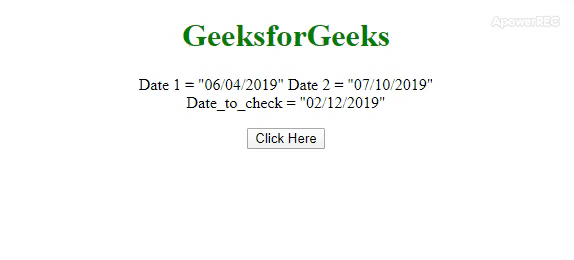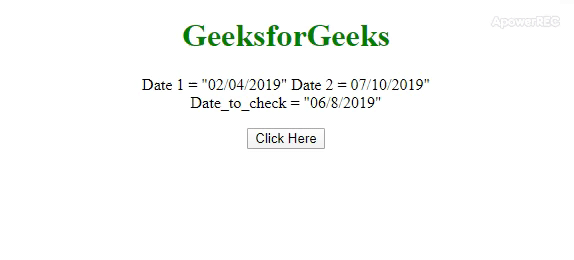# How to check if one date is between two dates in JavaScript ?

• Last Updated : 20 Jun, 2022

The task is to determine if the given date is in between the given 2 dates or not? Here are a few of the most used techniques discussed with the help of JavaScript. In the first approach, we will use .split() method and the new Date() constructor. And in the second approach we will use the .getTime() method with the new Date() constructor. Approach 1: Use .split() method to split the date on “/” to get the day, month and year in an array. The we have to construct the date from the array obtained in previous step for that we will use the new Date() constructor . Because this method returns the number of seconds from 1 Jan 1970, So it becomes easy to compare the dates.

• Example: This example uses the approach discussed above.

## html

 `` `<``html``>`   `<``head``>` `    ``<``title``>` `        ``How to Check if one Date is between` `        ``two dates using JavaScript ?` `    ``` `    `  `    ``<``style``>` `        ``body {` `            ``text-align: center;` `        ``}` `        ``h1 {` `            ``color: green;` `        ``}` `        ``#geeks {` `            ``font-weight: bold;` `        ``}` `    ``` ``   `<``body``>` `    ``<``h1``>GeeksforGeeks` `    `  `    ``<``p``>` `        ``Date 1 = "06/04/2019"` `        ``Date 2 = "07/10/2019"` `        ``<``br``>Date_to_check = "02/12/2019"` `    ``` `    `  `    ``<``button` `onclick="gfg_Run()">` `        ``Click Here` `    ``` `    `  `    ``<``p` `id="geeks">` `    `  `    ``<``script``>` `        ``var el_down = document.getElementById("geeks");` `    `  `        ``// Format - MM/DD/YYYY` `        ``var Date_1 = "06/04/2019";` `        ``var Date_2 = "07/10/2019";` `        ``var Date_to_check = "02/12/2019";`   `        ``function gfg_Run() {` `            ``D_1 = Date_1.split("/");` `            ``D_2 = Date_2.split("/");` `            ``D_3 = Date_to_check.split("/");` `            `  `            ``var d1 = new Date(D_1, parseInt(D_1) - 1, D_1);` `            ``var d2 = new Date(D_2, parseInt(D_2) - 1, D_2);` `            ``var d3 = new Date(D_3, parseInt(D_3) - 1, D_3);` `            `  `            ``if (d3 > d1 && d3 < ``d2``) {` `                ``el_down.innerHTML = "Date is in between the "` `                                    ``+ "Date 1 and Date 2";` `            ``} else {` `                ``el_down.innerHTML = "Date is not in between "` `                                    ``+ "the Date 1 and Date 2";` `            ``}` `        ``}` `    ``` ``   ``

• Output:Approach 2: Here first use new Date() constructor and pass the string in it which makes a Date Object. The .getTime() method which returns the number of seconds from 1 Jan 1970 and Seconds can be easily compared.

• Example: This example uses the approach discussed above.

## html

 `` `<``html``>`   `<``head``>` `    ``<``title``>` `        ``How to Check if one Date is between` `        ``two dates using JavaScript ?` `    ``` `    `  `    ``<``style``>` `        ``body {` `            ``text-align: center;` `        ``}` `        ``h1 {` `            ``color: green;` `        ``}` `        ``#geeks {` `            ``font-weight: bold;` `        ``}` `    ``` ``   `<``body``>` `    ``<``h1``>GeeksforGeeks` `    `  `    ``<``p``>` `        ``Date 1 = "06/04/2019" ` `        ``Date 2 = "07/10/2019"` `        ``<``br``>Date_to_check = "02/8/2019"` `    ``` `    `  `    ``<``button` `onclick="gfg_Run()">` `        ``Click Here` `    ``` `    `  `    ``<``p` `id="geeks">` `    `  `    ``<``script``>` `        ``var el_down = document.getElementById("geeks");`   `        ``// Format - MM/DD/YYYY` `        ``var D1 = "06/04/2019";` `        ``var D2 = "07/10/2019";` `        ``var D3 = "02/8/2019";`   `        ``function gfg_Run() {` `            ``D1 = new Date(D1);` `            ``D2 = new Date(D2);` `            ``D3 = new Date(D3);` `            `  `            ``if (D3.getTime() <= D2.getTime()` `                ``&& D3.getTime() >= D1.getTime()) {` `                ``el_down.innerHTML = "Date is in between"` `                        ``+ " the Date 1 and Date 2"; ` `            ``} else {` `                ``el_down.innerHTML = "Date is not in"` `                    ``+ " between the Date 1 and Date 2";` `            ``}` `        ``}` `    ``` ``   ``

• Output:My Personal Notes arrow_drop_up
Recommended Articles
Page :